# Introduction to spatial referencing

Today's blog post was written by Alex Taylor, a developer on the Image Processing Toolbox team. Thanks, Alex!

In MATLAB, an image is typically represented as a numeric matrix. There are some naturally geometric image processing operations where a user may need to care about where an image is located in a coordinate system. The term "spatial referencing" refers to the process of defining where an image is located in some coordinate system. This blog post will explore spatial referencing and the various ways to accomplish the spatial referencing of image data in MATLAB and the Image Processing Toolbox.

### Contents

#### Background: The Intrinsic Coordinate System

There is a consistent, default coordinate system used in MATLAB and the Image Processing Toolbox. This coordinate system is used by default for all naturally geometric operations such as image display, and geometric transformations. You can see the default coordinate system by displaying a small image and observing where the pixels are positioned within the coordinate system of the axes.

I = reshape(9:-1:1,3,3);
figure;
imagesc(I);
grid on;
colormap gray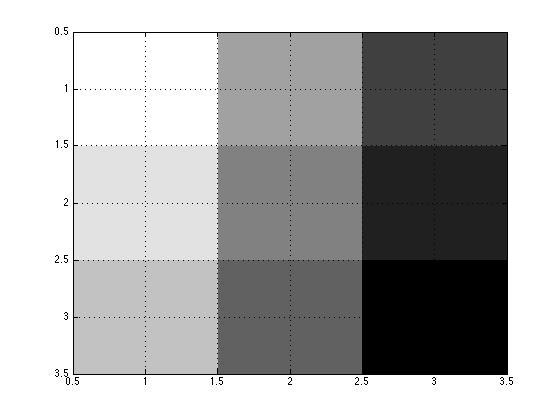In the default coordinate system, the center of each pixel falls on an integral value that corresponds to the one-based (column,row) subscripts of the image. For example, the center of the first pixel in I is located at coordinate (1.0,1.0).

In the Image Processing Toolbox, the default coordinate system is referred to as the "intrinsic coordinate system." The intrinsic coordinate system is a continuous extension of the discrete column,row subscripts of an image grid. This relationship between column,row matrix subscripting is where the term "intrinsic coordinates" comes from. The coordinate system is intrinsic to the one based column,row indexing of MATLAB.

#### Spatial referencing objects: imref2d and imref3d

The spatial referencing objects imref2d and imref3d were introduced in the R2013a release of the Image Processing Toolbox to make it easier to work with 2-D and 3-D images in a non-default or "world" coordinate system. This blog post will focus on the 2-D case and imref2d. The following example defines a world coordinate system for the simple image I where the X limits of the image are [3 6] and the Y limits the image are [6 9]. The X and Y world limits can be defined in any consistent unit system. In this example, assume the world limits are defined in units of meters.

RI = imref2d(size(I));
RI.XWorldLimits = [3 6];
RI.YWorldLimits = [6 9];


The equivalent spatial referencing object can be created in one line with a three-argument syntax.

RI = imref2d(size(I),[3 6],[6 9]);


Together, an image, I, and its associated spatial referencing object, RI, describe a spatially referenced image. The imshow function accepts a spatially referenced image.

figure, imshow(I,RI,[],'InitialMagnification','fit');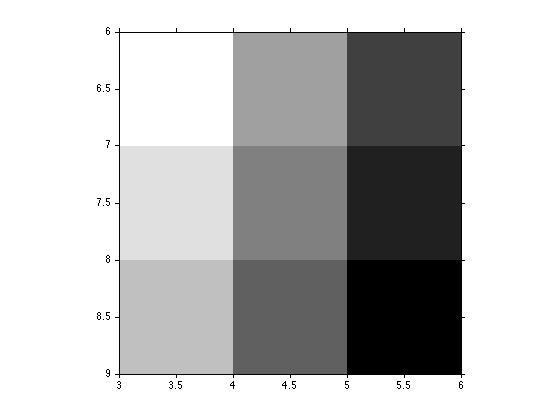#### Properties of imref2d

Spatial referencing objects have properties that describe various aspects of spatial referencing. The PixelExtentInWorldX and PixelExtentInWorldY properties define how large each pixel is in the world coordinate system along the X and Y dimensions. Because the XWorldLimits and YWorldLimits properties were defined in meters, the PixelExtentInWorldX and PixelExtentInWorldY properties are in units of meters.

RI.PixelExtentInWorldX
RI.PixelExtentInWorldY

ans =

1

ans =

1



The ImageExtentInWorldX and ImageExtentInWorldY properties define the size of the image grid along the X and Y dimensions in the units of the world coordinate system.

RI.ImageExtentInWorldX
RI.ImageExtentInWorldY

ans =

3

ans =

3



The ImageSize property defines the number of rows and columns in the associated image matrix.

RI.ImageSize

ans =

3     3



#### Converting between coordinate systems using spatial referencing objects

The spatial referencing objects have a set of related functions that can be used to convert from world coordinates to the default intrinsic coordinate system and to discrete pixel subscripts. For example, the worldToIntrinsic function can be used to find the intrinsic coordinates with the image I that correspond to the world (X,Y) coordinate (4.6,7.3).

[intrinsicX,intrinsicY] = worldToIntrinsic(RI,4.6,7.3)

intrinsicX =

2.1000

intrinsicY =

1.8000



The intrinsic coordinates that coorespond to this world coordinate pair fall at intrinsic (X,Y) location (2.1,1.8). This means that the value we are interested in is located between the first and second row and between that second and third column of the image I. The worldToSubscript function can be used to obtain the nearest integral row, column subscript into I at a given world location.

[r,c] = worldToSubscript(RI,4.6,7.3)

r =

2

c =

2



The output (row,column) location can be used to index into I to find the value of I at world (X,Y) location (4.6,7.3).

I(r,c)

ans =

5



The use of worldToSubscript to find the closest integrally valued subscript to a given world coordinate location is equivalent to performing nearest neighbor interpolation within the spatially referenced image grid.

interp2(I,intrinsicX,intrinsicY,'nearest')

ans =

5



You can also use bilinear interpolation to estimate the value of I at intrinsic coordinates that fall between integral row,column indices.

interp2(I,intrinsicX,intrinsicY,'bilinear')

ans =

4.9000



#### imshowpair and spatial referencing

The image display function imshowpair can be used to display overlays of image pairs and is a good example of the importance of spatial referencing in naturally geometric workflows.

Start with an MRI image of my knee after a running injury.

knee = dicomread('knee1.dcm');


Resize the knee image by a factor of 0.5.

smallKnee = imresize(knee,0.5);


Display a falsecolor overlay of each image. Note that because no spatial referencing information is specified to imshowpair, imshowpair assumes each image is in the intrinsic coordinate system. This causes each image to be aligned at the upper left corner (0.5,0.5).

figure, imshowpair(knee,smallKnee);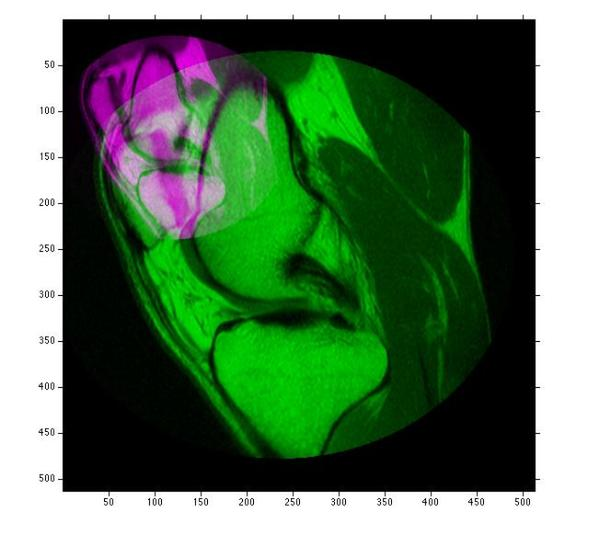There is a one input argument syntax of imref2d that allows for easy construction of the default spatial referencing that corresponds to an image of a given size. We can pass these spatial referencing objects to imshowpair to see the same visualization of each image overlayed in the intrinsic coordinate system.

Rknee    = imref2d(size(knee));
RsmallKnee = imref2d(size(smallKnee));
figure, imshowpair(knee,Rknee,smallKnee,RsmallKnee);In this case, the physical size of the knee in the world is the same, but one of the images is sampled at half the resolution of the other. Adjusting the world limits of the resized image has the effect of simulating a case in which two images of different resolution contain a view of the same scene in the world.

RsmallKnee.XWorldLimits = Rknee.XWorldLimits;
RsmallKnee.YWorldLimits = Rknee.YWorldLimits;
figure, imshowpair(knee,Rknee,smallKnee,RsmallKnee);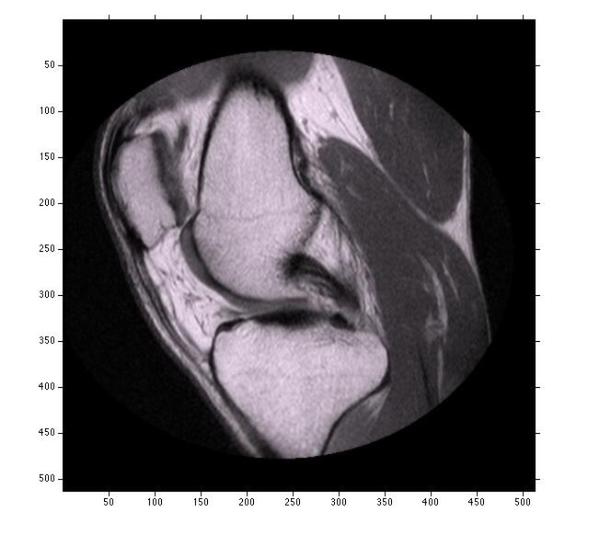Applying a shift to the world limits of one of the images simulates image misalignment due to translation.

RsmallKnee.XWorldLimits = RsmallKnee.XWorldLimits+50;
figure, imshowpair(knee,Rknee,smallKnee,RsmallKnee);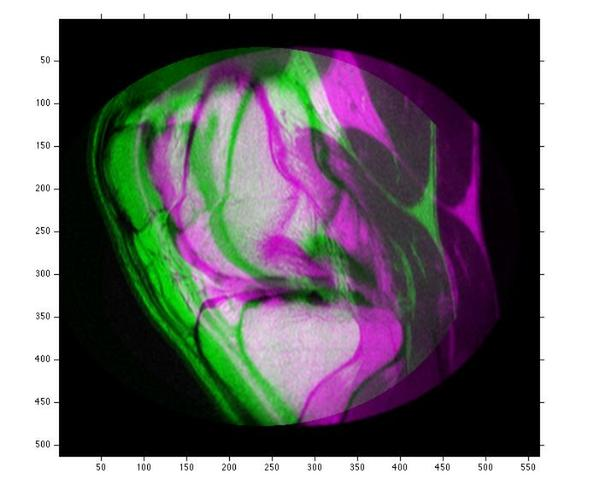#### The future direction of spatial referencing in the Image Processing Toolbox

Older Image Processing Toolbox functions such as imshow and imtransform use the the MATLAB graphics 'XData' and 'YData' referencing system. In this system, the two element vector XData described the centers of the first and last pixel along the X extent of the image, and the YData described the centers of the first and last pixel along the Y extent of the image. See the following doc link for details: The 'XData' and 'YData' properties of the HG image object.

Newer Image Processing processing functions such as imshowpair, imregister, imregtform, and imwarp use spatial referencing objects instead of 'XData' and 'YData'. Moving forward, we will de-emphasize the use of 'XData' and 'YData' referencing in the Image Processing Toolbox. We will expand the use of spatial refeferencing objects in new functions and find ways to integrate spatial referencing into existing functions that are part of naturally geometric workflows.

Are there any Image Processing Toolbox functions in which you would like to see the introduction of spatial referencing? If so, please let us know, we would appreciate your feedback.

Published with MATLAB® R2013a

|